Courses

# Olympiad Test: Fun With Magnets -1

## 20 Questions MCQ Test Science Olympiad Class 6 | Olympiad Test: Fun With Magnets -1

Description
This mock test of Olympiad Test: Fun With Magnets -1 for Class 6 helps you for every Class 6 entrance exam. This contains 20 Multiple Choice Questions for Class 6 Olympiad Test: Fun With Magnets -1 (mcq) to study with solutions a complete question bank. The solved questions answers in this Olympiad Test: Fun With Magnets -1 quiz give you a good mix of easy questions and tough questions. Class 6 students definitely take this Olympiad Test: Fun With Magnets -1 exercise for a better result in the exam. You can find other Olympiad Test: Fun With Magnets -1 extra questions, long questions & short questions for Class 6 on EduRev as well by searching above.
QUESTION: 1

Solution:
QUESTION: 2

Solution:
QUESTION: 3

### Which of the following can be attracted by a magnet?

Solution:
QUESTION: 4

Freely suspended magnet always comes to rest in the  ______ direction

Solution:
QUESTION: 5

The diagram below shows a simple pendulum facing a magnet XY. Attached to the pendulum is another magnet.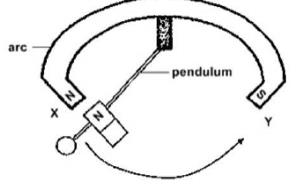Q. What forces are involved when the pendulum swings from X to Y?

Solution:
QUESTION: 6

Which of the following is used to make a permanent magnet?

Solution:
QUESTION: 7

If the north poles of two magnets are placed near one another, there is a:

Solution:
QUESTION: 8

Look at the diagram carefully.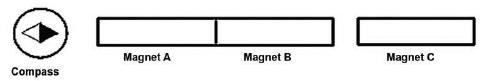When the three bar magnets are placed near each other, magnet A and B are attracted to each other but magnet B and C repel each other.
Q. Which of the following diagrams shows the correct poles for Magnet A and Magnet C?

Solution:
QUESTION: 9

A bar magnet is cut into four pieces. Which of the following observations would be true?

Solution:
QUESTION: 10

A bar of steel can be permanently magnetized by:

Solution:
QUESTION: 11

An artificial magnet which is used for finding geographical directions is called:

Solution:
QUESTION: 12

The surest test for magnetism is:

Solution:
QUESTION: 13

Shia had four magnets as shown below.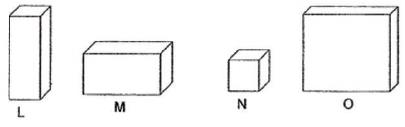She placed the magnets near a pile of staples and recorded her observation in the table below.
Magnet Distance between magnets and staples (cm) Number of staples attracted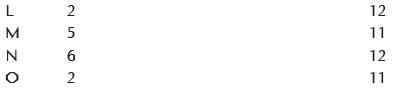Q. Which of the following about magnet is correct?

Solution:
QUESTION: 14

Alisha found three objects and wanted to test if they were magnets. She bought a magnet from the school bookshop and placed it next to each of the objects. This is what she observed.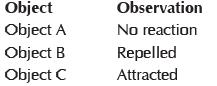Q. Which of the following object(s) is definitely a magnet?

Solution:
QUESTION: 15

A student tries to magnetize a short steel rod. Which of the following tests will show that she has succeeded?

Solution:
QUESTION: 16

There are following numbers of poles in a magnet:

Solution:

A magnet always has two poles: north pole and south pole

QUESTION: 17

Solution:
QUESTION: 18

The attraction of iron filings by the poles of a magnet is:

Solution:
QUESTION: 19

The presence of magnetism in a magnet is due to:

Solution:
QUESTION: 20

An effective length of a magnet is:

Solution: